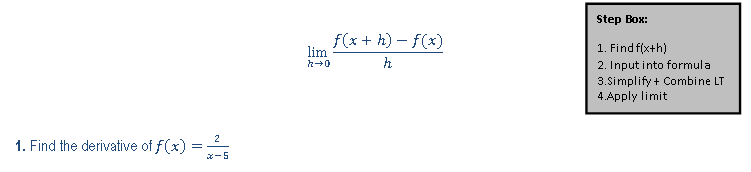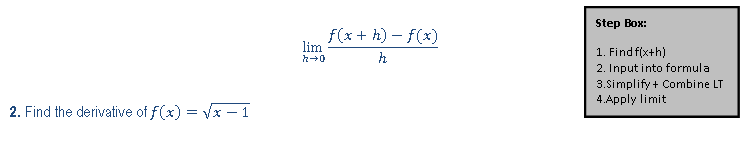Ch.6: Derivatives (Part 1)WorksheetSee all chapters
 Ch.1: Pre-Calc (Part 1) 3hrs & 31mins 0% complete Worksheet Ch.2: Pre-Calc (Part 2) 2hrs & 53mins 0% complete Worksheet Ch.3: Pre-Calc (Part 3) 1hr & 40mins 0% complete Worksheet Ch.4: Limits (Part 1) 2hrs & 25mins 0% complete Worksheet Ch.5: Limits (Part 2) 1hr & 53mins 0% complete Worksheet Ch.6: Derivatives (Part 1) 3hrs & 13mins 0% complete Worksheet Ch.7: Derivatives (Part 2) 2hrs & 26mins 0% complete Worksheet Ch.8: Applications of Derivatives (Part 1) 3hrs & 1min 0% complete Worksheet Ch.9: Applications of Derivatives (Part 2) 2hrs & 1min 0% complete Worksheet Ch.10: Applications of Derivatives (Part 3) 3hrs 0% complete Worksheet Ch.11: Integrals 3hrs & 8mins 0% complete Worksheet

# Intro to Derivative (Long Way)

See all sections
Sections
Intro to Derivative (Long Way)
Power Rule
Product Rule
Quotient Rule
Chain Rule

Concept #1: Definition of the Derivative

Concept #2: Intro

Practice: Find the derivative using the following equation,Practice: Find the derivative using the following equation: